Date: Mon, 25 Jan 1999 15:13:44 -0700 (MST) Sender: Roger How many cannonballs can be stacked in a triangular pyramid? Hi Roger If you stack cannonballs in a triangular pyramid then the top layer has one cannonball, the second layer has 3 cannonballs, the third layer has 6 cannonballs, ... These are triangular numbers. (See an earlier note in the Quandaries and Queries section called Triangular Numbers.) The nth triangular number is T(n) = n(n+1)/2 which you should recognize as the binomial coefficient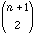which is the number of ways of selecting 2 things from n+1 things.    Thus the number of cannonballs in a triangular pyramid is 1 if the pyramid has 1 layer 1+3 if the pyramid has 2 layers 1+3+6 if the pyramid has 3 layers ... 1+3+6+...+n(n+1)/2 if the pyramid has n layers Hence the number of cannonballs in a triangular pyramid that is n layers high is T(1)+T(2)+T(3)+...+T(n).    There is a nice way to exprress this number which you can see as follows. Suppose that n+2 people are standing in a row and you are to walk down the row and select three of them. You can do this in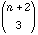ways.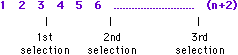The third person selected could be person number 3, person number 4, person nunber 5 ... up to person number (n+2). If the third person select is number 3 then the first two selected were 1 and 2 which could only be done in 1 = T(1) way. If the third person select is number 4 then the first two were selected from 1, 2, and 3 which could be done in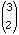= T(2) ways. If the third person select is number 5 then the first two were selected from 1, 2, 3 and 4 which could be done in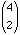= T(3) ways. ... If the third person select is number n+2 then the first two were selected from 1, 2, 3 ... n+1 which could be done in= T(n) ways. Thusthe number of ways of selecting 3 items from n+2 items is T(1)+T(2)+T(3)+...+T(n). Hence the number of cannonballs in a triangular pyramid that is n layers high is. Cheers Penny Go to Math Central To return to the previous page use your browser's back button.